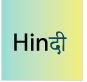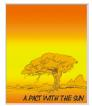Courses

# How to prepare for Class 6 Mathematics: Tips & Tricks for Mathematics Class 6 Notes | EduRev

Created by: Praveen Kumar

## Class 6 : How to prepare for Class 6 Mathematics: Tips & Tricks for Mathematics Class 6 Notes | EduRev

The document How to prepare for Class 6 Mathematics: Tips & Tricks for Mathematics Class 6 Notes | EduRev is a part of Class 6 category.
All you need of Class 6 at this link: Class 6

One thing to be kept in mind all along the way for Maths preparation is that practice is the key here. The more you practice for every topic, the better understanding is achieved.

The main topics in Class 6 Maths are basic arithmetic, algebra, geometry and mensuration, probability, etc. These topics should be practiced as much as possible. The key to solve problems is to by-heart the formulas, understand the theorems, and practice as many times as possible. Maths can become your favorite subject with practice and dedication.Mathematics Course Class 6- Notes, Questions, Videos & MCQs

Includes 68 docs, 222 videos & 26 tests

Tips & Tricks for Mathematics :

1. Study from the Mathematics NCERT Textbook and practice all the solved examples and exercise questions thoroughly before moving forward to extra practice from additional books. Refer to NCERT Solutions in case of any doubt.

## NCERT Textbooks and solutions

Chapter 1 - Knowing Our Numbers

Chapter 2 - Whole Numbers

Chapter 3 - Playing with Numbers

Chapter 4 - Basic Geometrical Ideas

Chapter 5 - Understanding Elementary Shapes

Chapter 6 - Integers

Chapter 7 - Fractions

Chapter 8 - Decimals

Chapter 9 - Data Handling

Chapter 10 - Mensuration

Chapter 11 - Algebra

Chapter 12 - Ratio and Proportion

Chapter 13 - Symmetry

Chapter 14 - Practical Geometry

2. Practice, above all. The range of questions in class 6 is limited. With substantial practice, most question types will be internalized, and you won’t have to think for most questions.

3. Develop a good understanding of topics. It will help you with those challenging questions or derive formulae in the rare chance you forget. This can be done by watching videos for various topics that will help you develop an interest in the topic.

4. Understand all the concepts in as much depth as you can. And then solve different varieties of questions and problems. Attempt various topic-wise and chapter-wise tests. Also, practice mre with sample papers and previous year papers. Use your knowledge to solve some real life questions as well

## Practice tests

5. Give more importance to the topics which have high weightage. Solve the previous year exam papers which will increase your confidence and give you a pre-exam feel. It also helps to brush up all your concepts before the exam.

6. Don’t just read or mug up the topics but practice them. This is the most important thing which you need to keep in mind as reading maths does not help you. Instead, make a routine and give some time for practice

## Important Topics and Preparation tips for Each Chapter

Chapter 1: Knowing our numbers

This is an introduction to the number system. Students get to understand and appreciate the meaning of the words thousand, lakhs, millions etc, and are expected to know how to write big denominations of numbers and count them in this chapter in 6th class maths. They learn the Indian system of counting and the International system of counting.

Chapter 2: Whole numbers

This introduces students to natural, whole, non-decimal numbers. It introduces the concepts of predecessors and successors in the number system.

Chapter 3: Playing with numbers

This is an introduction for students to the concept of factors of numbers, multiples of numbers etc.

Chapter 4: Basic geometrical ideas

This introduces students to the concepts of point, line, rays, segments etc.

Chapter 5: Understanding elementary shapes

This is an introduction to basic shapes like circle, triangles, rectangles, squares etc.

Chapter 6: Integers

This chapter is about conceptualizing the number line and the positions of the numbers on the same. It enables students to have a vision about which number lies where which is more or which is less etc. This is an important chapter in 6th class maths.

Chapter 7: Fractions

This chapter introduces the concepts of fractions as parts of a whole.

Chapter 8: Decimals

This is an introduction to the concept of point and decimals, and their relationship to fractions.

Chapter 9: Data handling

This shows students how to arrange numbers or items in a list according to a specified order using tally marks. It is a prerequisite to study statistics.

Chapter 10: Mensuration

Teaches concepts of perimeter and areas of simple geometric figures.

Chapter 11: Ratio and proportion

Building on the previous concepts of fractions, this chapter prepares the student to see ratios and proportions as relationships of parts with respect to the whole.

Chapter 12: Algebra

This introduces students to the concepts of algebra; finding out the value of an unknown quantity using what information is known, or assessing the relationship between unknown variables using known variables.

Chapter 13: Symmetry

This chapter builds on the conception and understanding of geometrical figures and shapes to introduce the concept of symmetry to students. Students are given

incomplete figures and are expected to make them symmetric, or identify how given figures are symmetric or not, etc.

Chapter 14: Practical geometry

This chapter is an introduction to how to draw basic geometric shapes using the geometric equipment. Students are given specific instructions and are expected to follow the same to draw the figures. For example: – draw two circles of radii 2.5 cm and 4 cm which have a common centre. Students are expected to measure these radii on their scales and use their compasses to draw these circles to complete the exercise.

## Other Subjects of Class 6:Social Science Studies (SST) Class 6 (VI) - CBSE, NCERT

Includes 103 docs, 66 videos & 58 testsScience for Class 6 (VI) - CBSE & NCERT Curriculum

Includes 90 docs, 90 videos & 32 testsEnglish for Class 6 (VI) - CBSE and NCERT Curriculum

Includes 48 docsHindi (Vasant) for Class 6 (VI) - CBSE and NCERT Curriculum

Includes 46 docs & 30 videosEnglish (A Pact with the Sun) for Class 6 (VI)

Includes 20 docs & 53 videos

,

,

,

,

,

,

,

,

,

,

,

,

,

,

,

,

,

,

,

,

,

,

,

,

,

,

;